Customers often ask questions about how to sort the rows of a table or a crosstab by a specific column, when the column is chosen dynamically according to a certain criteria or specified directly by the end user. This can be trivially accomplished for a table, because the exact number and names of the individual columns is previously known and fixed. In most cases it is simply a matter of specifying an appropriate sorting expression to the table. On the other hand, sorting a crosstab by a specific column is not so simple to accomplish. The crosstab generates its rows and columns dynamically from the provided data source, according to the specified row and column groups, and the crosstab body contains only aggregated data. Just sorting the records of the data source would accomplish nothing. It is necessary to sort the individual row groups of the crosstab according to the aggregated values in the crosstab body cells for the given column. To make things more clear, let us illustrate the problem with a simple example. The example runs against the “Adventure Works” sample database that comes with every installation of Telerik Reporting.

Dynamic sorting of tables

Let us consider the following SQL select statement against the “Adventure Works” sample database:

`SELECT` `Product.``Name` `AS` `ProductName, `
`    ``Product.ProductNumber ``AS` `ProductNumber, `
`    ``ProductCategory.``Name` `AS` `CategoryName, `
`    ``ProductSubcategory.``Name` `AS` `SubcategoryName `
`FROM   ``Production.Product ``AS` `Product `
`    ``JOIN` `Production.ProductSubcategory ``AS` `ProductSubcategory `
`       ``ON` `ProductSubcategory.ProductSubcategoryID = `
`         ``Product.ProductSubcategoryID `
`    ``JOIN` `Production.ProductCategory ``AS` `ProductCategory `
`       ``ON` `ProductCategory.ProductCategoryID = `
`         ``ProductSubcategory.ProductCategoryID`

The above SQL query lists the available products and their corresponding categories and subcategories: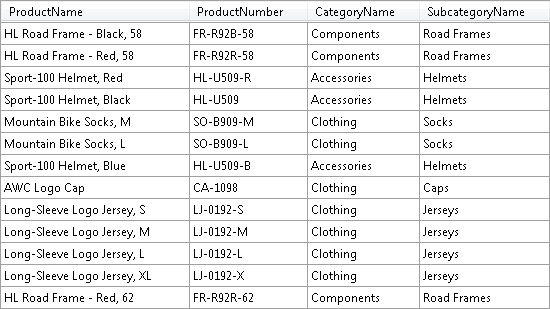With the help of Table Wizard it is fairly easy to turn this SQL statement into a tabular report that displays information about the available products.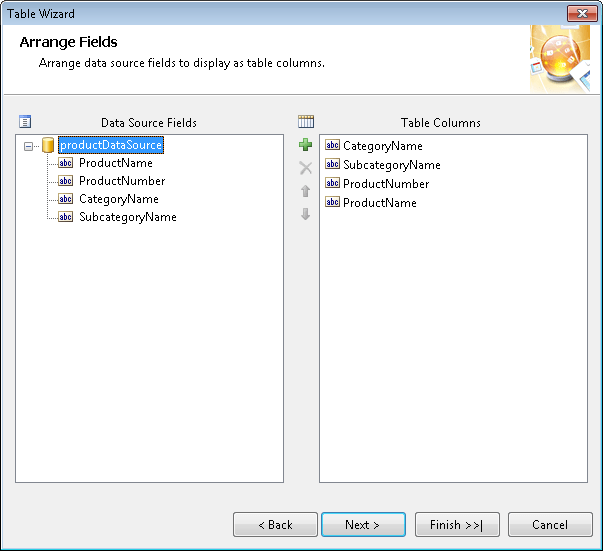Now let us define a report parameter called SortColumn that allows the user to specify the name of the column by which to sort the data. To apply the actual sorting criteria to the table, according to the current value of the report parameter, we can use the following expression:

`=Fields(Parameters.SortColumn.Value)`

The above statement uses the notation “Fields(field name)” that allows resolving the name of a field on the fly from a string expression. For example, if the value of the SortColumn parameter is “CategoryName”, the previous expression is equivalent to the following one:

`=Fields.CategoryName`

This effectively sorts the table rows by the CategoryName column. You can apply even more sophisticated sorting criteria using this or similar approaches, as long as the table columns are derived directly from the data source fields. Tables with dynamic columns generated from column groups cannot be sorted this way however.

Dynamic sorting of crosstabs

Let us extend the previous example by adding the orders for each product to our SQL query:

`SELECT` `Product.``Name` `AS` `ProductName, `
`    ``Product.ProductNumber ``AS` `ProductNumber, `
`    ``ProductCategory.``Name` `AS` `CategoryName, `
`    ``ProductSubcategory.``Name` `AS` `SubcategoryName, `
`    ``OrderHeader.OrderDate ``AS` `OrderDate, `
`    ``OrderDetail.LineTotal ``AS` `LineTotal `
`FROM   ``Production.Product ``AS` `Product `
`    JOIN` `Production.ProductSubcategory ``AS` `ProductSubcategory `
`       ON` `ProductSubcategory.ProductSubcategoryID = `
`         Product.ProductSubcategoryID `
`    ``JOIN` `Production.ProductCategory ``AS` `ProductCategory `
`       ``ON` `ProductCategory.ProductCategoryID = `
`         ``ProductSubcategory.ProductCategoryID `
`    ``JOIN` `Sales.SalesOrderDetail ``AS` `OrderDetail `
`       ``ON` `OrderDetail.ProductID = `
`         ``Product.ProductID `
`    ``JOIN` `Sales.SalesOrderHeader ``AS` `OrderHeader `
`       ``ON` `OrderHeader.SalesOrderID = `
`         ``OrderDetail.SalesOrderID `

The above SQL query produces a result set similar to the one shown in the following figure: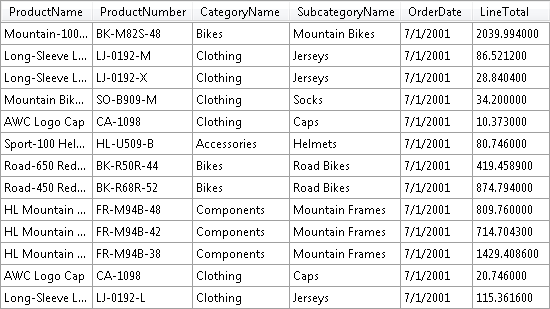With the help of Crosstab Wizard we can create a simple crosstab report that displays the sum of product sales grouped by category and subcategory per each year. The actual crosstab layout in this example is as follows:

• Column groups:
• OrderDate.Year
• Row groups:
• CategoryName
• SubcategoryName
• Detail values:
• Sum(LineTotal)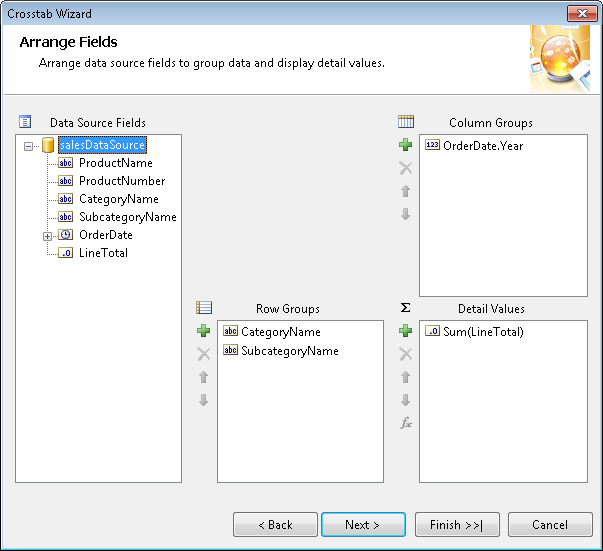The generated crosstab report looks similar to the one shown in the following figure: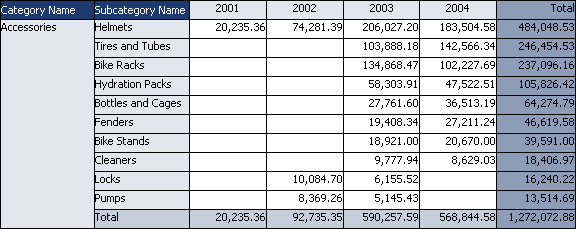Now let us define a report parameter called SortYear that allows the user to choose a year in the range between 2001 and 2004. If the user chooses the year 2003 for example, how can we sort the individual subcategories in each product category according to the sales for that year? The first naïve attempt is to apply a sorting expression like the following one to the SubcategoryName crosstab row group:

`=Sum``(Fields.LineTotal)`

The above expression does not quite work as expected however. Since the Sum aggregate function operates on product subcategory level, it sums all product sales for all years of the current subcategory. This effectively sorts the crosstab by the last total column regardless of the chosen year. What we really need is to filter somehow the input values passed to the Sum aggregate function to only those that apply to the chosen year. To accomplish this we need to define a custom aggregate function that sums only the values that satisfy a certain filtering criteria.

To define a custom aggregate function we need to create a new class that implements the IAggregateFunction interface. The IAggregateFunction interface has several methods we need to implement:

• Init – this method is called by the reporting engine every time the accumulation of the aggregate function must start over for a new subset of data. This is the right place to reset the currently accumulated value.
• Accumulate – this method is called for each new record of the accumulated subset of data. The method accepts an array of values as its only parameter. Each element of the array corresponds to a single argument of the aggregate function. This allows us to define aggregate functions with an arbitrary number of arguments.
• Merge – this method is called when the reporting engine needs to merge the results of two aggregate functions of the same type applied to different subsets of data.
• GetValue – this method must return the currently accumulated value by the aggregate function.

The following source code below defines a custom aggregate function called ConditionalSum:

C#

`/// <summary> `
`/// Defines an aggregate function to sum all numeric values that satisfy `
`/// a certain boolean filtering condition. `
`/// </summary> `
`/// <remarks> `
`/// This aggregate function accepts two arguments: `
`/// number - a numeric value to accumulate to the aggregate function; `
`/// filter - a boolean filtering condition that must be satisfied in `
`/// order to accumulate the current number to the aggregate function; `
`/// </remarks> `
`public` `class` `ConditionalSum : IAggregateFunction `
`{ `
`    ``// Contains the currently accumulated value of the aggregate function. `
`    ``double` `sum; `
` `
`    ``/// <summary> `
`    ``/// Initializes the current aggregate function to its initial state `
`    ``/// ready to accumulate and merge values.  `
`    ``/// </summary> `
`    ``/// <remarks> `
`    ``/// This method is called every time the accumulation of values must `
`    ``/// start over for a new subset of records from the data source. `
`    ``/// </remarks> `
`    ``public` `void` `Init() `
`    ``{ `
`        ``this``.sum = 0; `
`    ``} `
` `
`    ``/// <summary> `
`    ``/// Accumulates new argument values to the current aggregate function. `
`    ``/// </summary> `
`    ``/// <param name="values"> `
`    ``/// Specifies an array of argument values for the aggregate function. `
`    ``/// </param> `
`    ``/// <remarks> `
`    ``/// This aggregate function accepts two arguments: `
`    ``/// number - a numeric value to accumulate to the aggregate function; `
`    ``/// filter - a boolean filtering condition that must be satisfied in `
`    ``/// order to accumulate the current number to the aggregate function; `
`    ``/// </remarks> `
`    ``public` `void` `Accumulate(``object``[] values) `
`    ``{ `
`        ``// Extract the number argument from the specified array. `
`        ``double` `number = Convert.ToDouble(values); `
`        ``// Extract the filter argument from the specified array. `
`        ``bool` `filter = Convert.ToBoolean(values); `
` `
`        ``// Accumulate the value only if the condition is satisfied. `
`        ``if` `(filter) `
`        ``{ `
`            ``this``.sum += number; `
`        ``} `
`    ``} `
` `
`    ``/// <summary> `
`    ``/// Merges the specified aggregate function to the current one. `
`    ``/// </summary> `
`    ``/// <param name="aggregate"> `
`    ``/// Specifies an aggregate function to be merged to the current one. `
`    ``/// </param> `
`    ``/// <remarks> `
`    ``/// This method allows the reporting engine to merge two accumulated `
`    ``/// subsets of the same aggregate function into a single result. `
`    ``/// </remarks> `
`    ``public` `void` `Merge(IAggregateFunction aggregate) `
`    ``{ `
`        ``// Accumulate the value of the specified aggregate function. `
`        ``this``.sum += Convert.ToDouble(aggregate.GetValue()); `
`    ``} `
` `
`    ``/// <summary> `
`    ``/// Returns the currently accumulated value of the aggregate function. `
`    ``/// </summary> `
`    ``/// <returns> `
`    ``/// The currently accumulated numeric value of the aggregate function. `
`    ``/// </returns> `
`    ``public` `object` `GetValue() `
`    ``{ `
`        ``return` `this``.sum; `
`    ``} `
`} `

VB

`''' <summary> `
`''' Defines an aggregate function to sum all numeric values that satisfy `
`''' a certain boolean filtering condition. `
`''' </summary> `
`''' <remarks> `
`''' This aggregate function accepts two arguments: `
`''' number - a numeric value to accumulate to the aggregate function; `
`''' filter - a boolean filtering condition that must be satisfied in `
`''' order to accumulate the current number to the aggregate function; `
`''' </remarks> `
`Public` `Class` `ConditionalSum `
`    ``Implements` `IAggregateFunction `
` `
`    ``' Contains the currently accumulated value of the aggregate function. `
`    ``Public` `Sum ``As` `Double`
` `
`    ``''' <summary> `
`    ``''' Initializes the current aggregate function to its initial state `
`    ``''' ready to accumulate and merge values.  `
`    ``''' </summary> `
`    ``''' <remarks> `
`    ``''' This method is called every time the accumulation of values must `
`    ``''' start over for a new subset of records from the data source. `
`    ``''' </remarks> `
`    ``Public` `Sub` `Init() ``_`
`        ``Implements IAggregateFunction.Init `
` `
`        ``Me``.Sum = 0 `
`    ``End` `Sub`
` `
`    ``''' <summary> `
`    ``''' Accumulates new argument values to the current aggregate function. `
`    ``''' </summary> `
`    ``''' <param name="Values"> `
`    ``''' Specifies an array of argument values for the aggregate function. `
`    ``''' </param> `
`    ``''' <remarks> `
`    ``''' This aggregate function accepts two arguments: `
`    ``''' number - a numeric value to accumulate to the aggregate function; `
`    ``''' filter - a boolean filtering condition that must be satisfied in `
`    ``''' order to accumulate the current number to the aggregate function; `
`    ``''' </remarks> `
`    ``Public` `Sub` `Accumulate(``ByVal` `Values() ``As` `Object``) _ `
`        ``Implements` `IAggregateFunction.Accumulate `
` `
`        ``' Extract the number argument from the specified array. `
`        ``Dim` `Number ``As` `Double` `= Convert.ToDouble(Values(0)) `
`        ``' Extract the filter argument from the specified array. `
`        ``Dim` `Filter ``As` `Boolean` `= Convert.ToBoolean(Values(1)) `
` `
`        ``' Accumulate the value only if the condition is satisfied. `
`        ``If` `Filter ``Then`
`            ``Me``.Sum = ``Me``.Sum + Number `
`        ``End` `If`
`    ``End` `Sub`
` `
`    ``''' <summary> `
`    ``''' Merges the specified aggregate function to the current one. `
`    ``''' </summary> `
`    ``''' <param name="Aggregate"> `
`    ``''' Specifies an aggregate function to be merged to the current one. `
`    ``''' </param> `
`    ``''' <remarks> `
`    ``''' This method allows the reporting engine to merge two accumulated `
`    ``''' subsets of the same aggregate function into a single result. `
`    ``''' </remarks> `
`    ``Public` `Sub` `Merge(``ByVal` `Aggregate ``As` `IAggregateFunction) _ `
`        ``Implements` `IAggregateFunction.Merge `
` `
`        ``' Accumulate the value of the specified aggregate function. `
`        ``Me``.Sum = ``Me``.Sum + Convert.ToDouble(Aggregate.GetValue()) `
`    ``End` `Sub`
` `
`    ``''' <summary> `
`    ``''' Returns the currently accumulated value of the aggregate function. `
`    ``''' </summary> `
`    ``''' <returns> `
`    ``''' The currently accumulated numeric value of the aggregate function. `
`    ``''' </returns> `
`    ``Public` `Function` `GetValue() ``As` `Object` `_ `
`        ``Implements` `IAggregateFunction.GetValue `
` `
`        ``Return` `Me``.Sum `
`    ``End` `Function`
`End` `Class`

The ConditionalSum aggregate function accepts two arguments: the first one is a numeric value to be accumulated to the sum; the second one is a boolean filtering expression. The aggregate function checks the result of the filtering expression for each new value: if the expression evaluates to true – the current value is accumulated to the sum; if the expression evaluates to false – the current value is skipped. This allows us to define a sorting expression like the following one:

`=ConditionalSum(Fields.LineTotal, `
`                Fields.OrderDate.Year` `= `
`                Parameters.SortYear.Value)`

The above expression computes the sum of the product sales where the current year is equal to the value of the SortYear report parameter. Applying this expression as sorting criteria to the SubcategoryName row group will produce the desired result: sorting the crosstab according to the chosen year.

You can download the sample project attached to this post that illustrates the discussed technique. You need the "Adventure Works" sample database in order to run this sample on your computer. If the application fails to connect to the database for some reason, please make sure that the connection string in the App.config file is specified correctly.